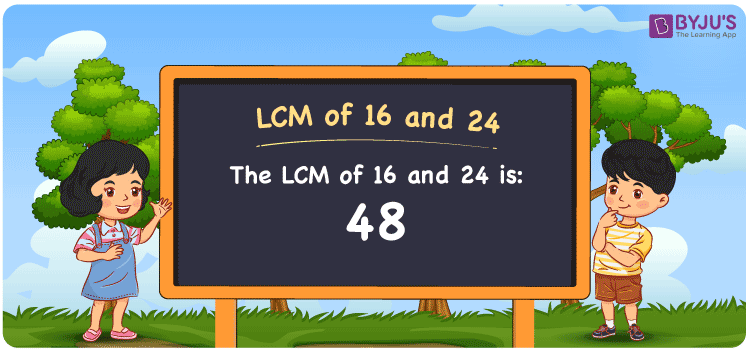Checkout JEE MAINS 2022 Question Paper Analysis : Checkout JEE MAINS 2022 Question Paper Analysis :

# LCM of 16 and 24

LCM of 16 and 24 is 48. The LCM is the common multiple which is evenly divisible by the two given numbers. Least common multiple of 16 and 24 is the smallest number which we obtain from the common multiples. (16, 32, 48, 64, 80, 96, ….) and (24, 48, 72, 96, 120, 144,….) are the first few multiples of 16 and 24. Certain methods like division, prime factorisation and listing of multiples can be used to find the LCM.

## What is LCM of 16 and 24?

The answer to this question is 48. The LCM of 16 and 24 using various methods is shown in this article for your reference. The LCM of two non-zero integers, 16 and 24, is the smallest positive integer 48 which is divisible by both 16 and 24 with no remainder.## How to Find LCM of 16 and 24?

LCM of 16 and 24 can be found using three methods:

• Prime Factorisation
• Division method
• Listing the multiples

### LCM of 16 and 24 Using Prime Factorisation Method

The prime factorisation of 16 and 24, respectively, is given by:

16 = 2 x 2 x 2 x 2 = 2

24 = 2 x 2 x 2 x 3 = 2³ x 3¹

LCM (16, 24) = 48

### LCM of 16 and 24 Using Division Method

We’ll divide the numbers (16, 24) by their prime factors to get the LCM of 16 and 24 using the division method (preferably common). The LCM of 16 and 24 is calculated by multiplying these divisors.

 2 16 24 2 8 12 2 4 6 2 2 3 3 1 3 x 1 1

No further division can be done.

Hence, LCM (16, 24) = 48

### LCM of 16 and 24 Using Listing the Multiples

To calculate the LCM of 16 and 24 by listing out the common multiples, list the multiples as shown below

 Multiples of 16 Multiples of 24 16 24 32 48 48 72 64 96 80 120

LCM (16, 24) = 48

## Video Lesson on Applications of LCM## LCM of 16 and 24 Solved Examples

What is the LCM if the product of two numbers is 384 and the GCD is 8?

Solution:

It is given that

Product of two numbers = 384

GCD = 8

We know that

LCM x GCD = Product of two numbers

LCM = Product/GCD

LCM = 384/8

LCM = 48

Hence, the LCM is 48.

## Frequently Asked Questions on LCM of 16 and 24

### Find the LCM of 16 and 24.

The LCM of 16 and 24 is 48. To find the LCM, the multiples of 16 and 24 should be found and the smallest multiple that is exactly divisible by 16 and 24 has to be determined.

### Which methods can be used to find the LCM of 16 and 24?

The methods that can be used to find the LCM of 16 and 24 are Prime Factorization Method, Division Method and Listing multiples.

### Calculate the GCF if the LCM of 16 and 24 is 48.

LCM x GCF = 16 x 24

Given

LCM of 16 and 24 = 48

48 x GCF = 384

GCF = 384/48 = 8